This notebook contains an excerpt from the Python Data Science Handbook by Jake VanderPlas; the content is available on GitHub.

The text is released under the CC-BY-NC-ND license, and code is released under the MIT license. If you find this content useful, please consider supporting the work by buying the book!

No changes were made to the contents of this notebook from the original.

# Density and Contour Plots¶

Sometimes it is useful to display three-dimensional data in two dimensions using contours or color-coded regions. There are three Matplotlib functions that can be helpful for this task: plt.contour for contour plots, plt.contourf for filled contour plots, and plt.imshow for showing images. This section looks at several examples of using these. We'll start by setting up the notebook for plotting and importing the functions we will use:

In :
%matplotlib inline
import matplotlib.pyplot as plt
plt.style.use('seaborn-white')
import numpy as np


## Visualizing a Three-Dimensional Function¶

We'll start by demonstrating a contour plot using a function $z = f(x, y)$, using the following particular choice for $f$ (we've seen this before in Computation on Arrays: Broadcasting, when we used it as a motivating example for array broadcasting):

In :
def f(x, y):
return np.sin(x) ** 10 + np.cos(10 + y * x) * np.cos(x)


A contour plot can be created with the plt.contour function. It takes three arguments: a grid of x values, a grid of y values, and a grid of z values. The x and y values represent positions on the plot, and the z values will be represented by the contour levels. Perhaps the most straightforward way to prepare such data is to use the np.meshgrid function, which builds two-dimensional grids from one-dimensional arrays:

In :
x = np.linspace(0, 5, 50)
y = np.linspace(0, 5, 40)

X, Y = np.meshgrid(x, y)
Z = f(X, Y)


Now let's look at this with a standard line-only contour plot:

In :
plt.contour(X, Y, Z, colors='black');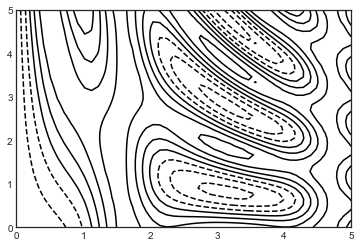Notice that by default when a single color is used, negative values are represented by dashed lines, and positive values by solid lines. Alternatively, the lines can be color-coded by specifying a colormap with the cmap argument. Here, we'll also specify that we want more lines to be drawn—20 equally spaced intervals within the data range:

In :
plt.contour(X, Y, Z, 20, cmap='RdGy');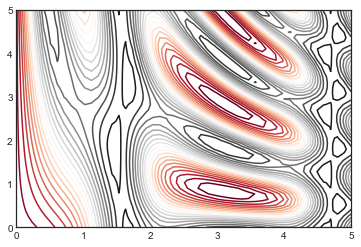Here we chose the RdGy (short for Red-Gray) colormap, which is a good choice for centered data. Matplotlib has a wide range of colormaps available, which you can easily browse in IPython by doing a tab completion on the plt.cm module:

plt.cm.<TAB>

Our plot is looking nicer, but the spaces between the lines may be a bit distracting. We can change this by switching to a filled contour plot using the plt.contourf() function (notice the f at the end), which uses largely the same syntax as plt.contour().

Additionally, we'll add a plt.colorbar() command, which automatically creates an additional axis with labeled color information for the plot:

In :
plt.contourf(X, Y, Z, 20, cmap='RdGy')
plt.colorbar();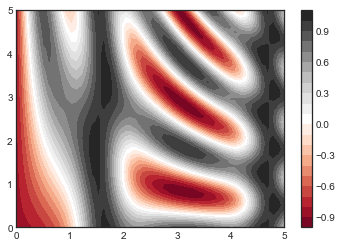The colorbar makes it clear that the black regions are "peaks," while the red regions are "valleys."

One potential issue with this plot is that it is a bit "splotchy." That is, the color steps are discrete rather than continuous, which is not always what is desired. This could be remedied by setting the number of contours to a very high number, but this results in a rather inefficient plot: Matplotlib must render a new polygon for each step in the level. A better way to handle this is to use the plt.imshow() function, which interprets a two-dimensional grid of data as an image.

The following code shows this:

In :
plt.imshow(Z, extent=[0, 5, 0, 5], origin='lower',
cmap='RdGy')
plt.colorbar()
plt.axis(aspect='image');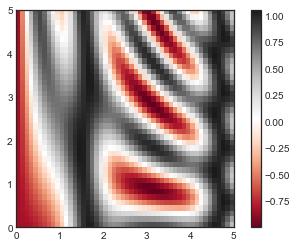There are a few potential gotchas with imshow(), however:

• plt.imshow() doesn't accept an x and y grid, so you must manually specify the extent [xmin, xmax, ymin, ymax] of the image on the plot.
• plt.imshow() by default follows the standard image array definition where the origin is in the upper left, not in the lower left as in most contour plots. This must be changed when showing gridded data.
• plt.imshow() will automatically adjust the axis aspect ratio to match the input data; this can be changed by setting, for example, plt.axis(aspect='image') to make x and y units match.

Finally, it can sometimes be useful to combine contour plots and image plots. For example, here we'll use a partially transparent background image (with transparency set via the alpha parameter) and overplot contours with labels on the contours themselves (using the plt.clabel() function):

In :
contours = plt.contour(X, Y, Z, 3, colors='black')
plt.clabel(contours, inline=True, fontsize=8)

plt.imshow(Z, extent=[0, 5, 0, 5], origin='lower',
cmap='RdGy', alpha=0.5)
plt.colorbar();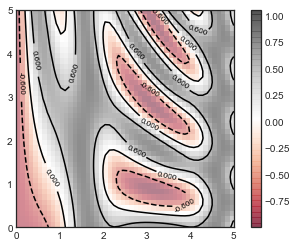The combination of these three functions—plt.contour, plt.contourf, and plt.imshow—gives nearly limitless possibilities for displaying this sort of three-dimensional data within a two-dimensional plot. For more information on the options available in these functions, refer to their docstrings. If you are interested in three-dimensional visualizations of this type of data, see Three-dimensional Plotting in Matplotlib.